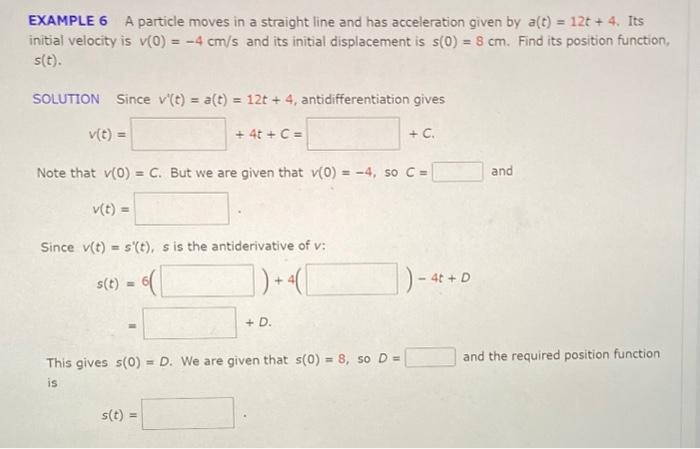Home / Expert Answers / Calculus / example-6-a-particle-moves-in-a-straight-line-and-has-acceleration-given-by-a-t-12t-4-its-initia-pa628

# (Solved): EXAMPLE 6 A particle moves in a straight line and has acceleration given by a(t) = 12t+4. Its initia ...

EXAMPLE 6 A particle moves in a straight line and has acceleration given by a(t) = 12t+4. Its initial velocity is v(0) = -4 cm/s and its initial displacement is s(0) = 8 cm. Find its position function, s(t). SOLUTION Since v'(t) = a(t) = 12t+4, antidifferentiation gives v(t) = Note that v(0) = C. But we are given that v(0) = -4, so C = v(t) = Since v(t) = s'(t), s is the antiderivative of v: |) + 4( [ s(t) = = + 4t + C = ( s(t) = + D. This gives s(0) = D. We are given that s(0) = 8, so D = is + C. - 4t+ D and and the required position functionEXAMPLE 6 A particle moves in a straight line and has acceleration given by . Its initial velocity is and its initial displacement is . Find its position function, . SOLUTION Since , antidifferentiation gives Note that . But we are given that , so and Since , is the antiderivative of : This gives . We are given that , so and the required position function is

We have an Answer from Expert